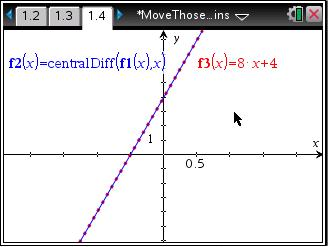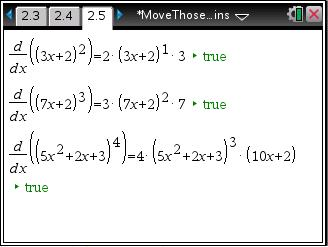# Activities

••• ##### Subject Area

• Math: Calculus: Derivatives

• ##### Author9-12

15 Minutes

• ##### Device
•TI-Nspire™ CX/CX II
•TI-Nspire™ CX CAS/CX II CAS
• TI-Nspire™
• TI-Nspire™ CAS
• ##### Software

TI-Nspire™
TI-Nspire™ CAS

3.2

## Move Those Chains

#### Activity Overview

In this activity, students will explore the Chain Rule. Students are asked to make a conjecture of the derivative of f(x) = (2x + 1)2 based on the Power Rule. They are then asked to graph their derivative function and compare it to the graph of (x). They will then examine "true" statements about various derivatives of composite functions. They will observe patterns create a rule for finding the derivative of other composite functions.

#### Key Steps

•In this activity, students will explore the Chain Rule. Students are first asked to make a conjecture of the derivative of a composite function based on the Power Rule. They are then asked to graph their derivative function and compare it to the graph of the actual derivative.

•Students will then examine "true" statements about various derivatives of composite functions and use these patterns to create a rule for finding the derivative of other composite functions. They will then use their rule to create “true” examples of their own.